## Mathematical Modeling Products

#### Mathematical Modeling Resources (Grades 3-5)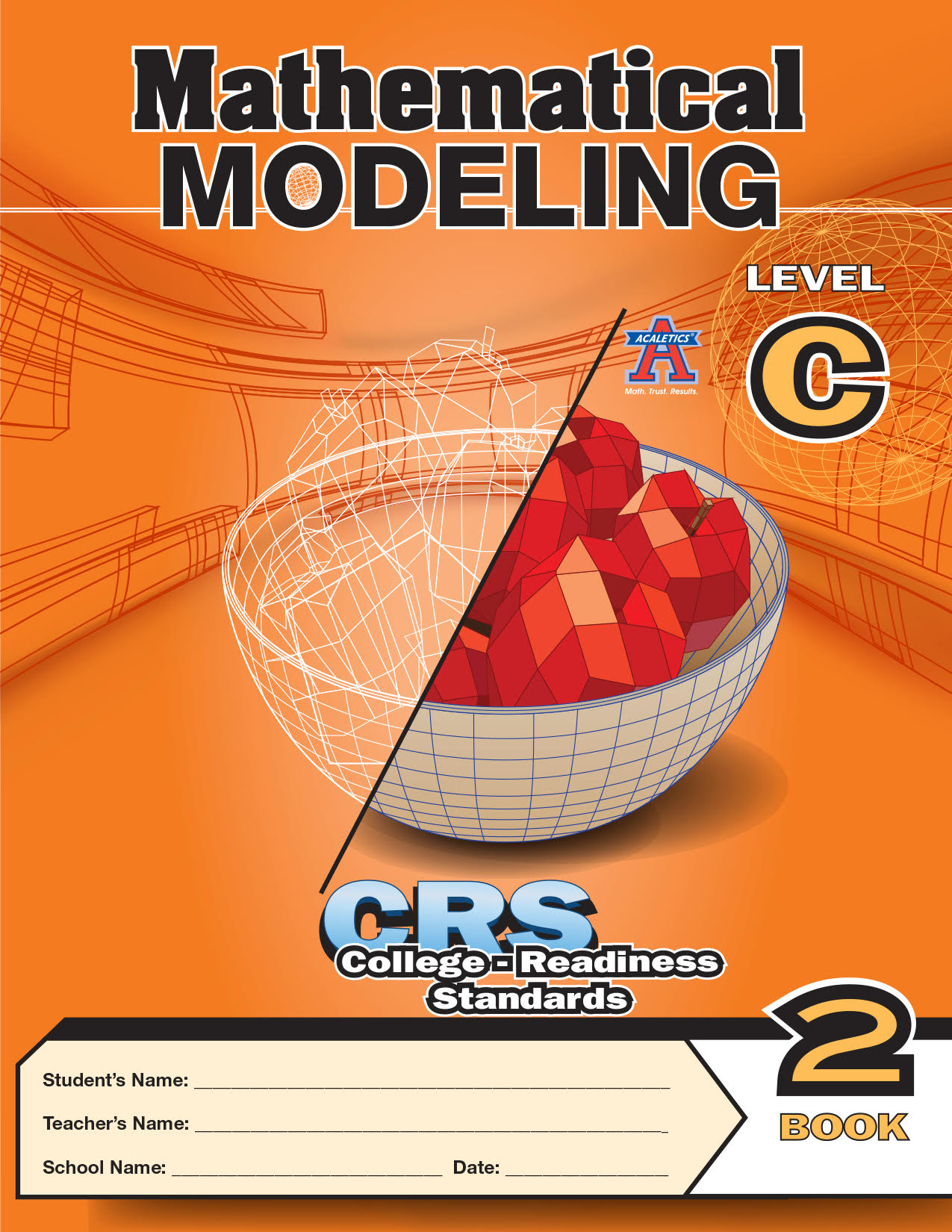close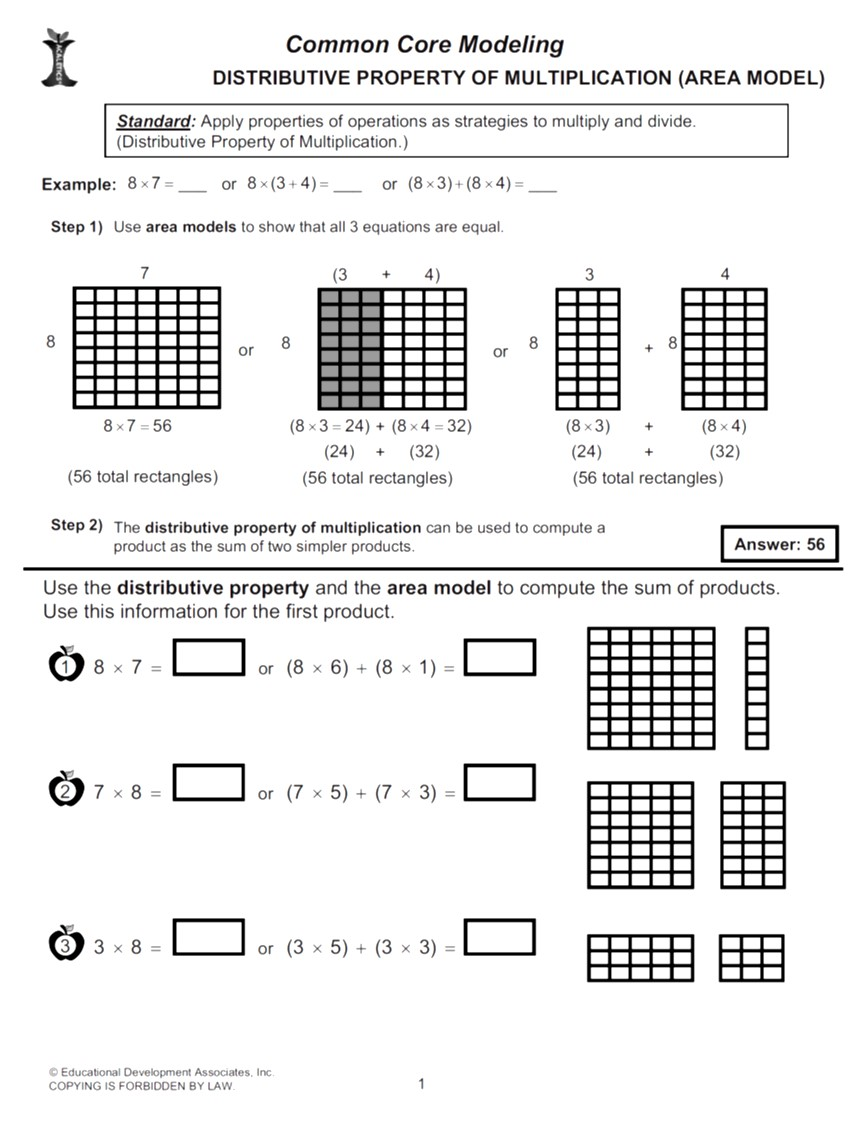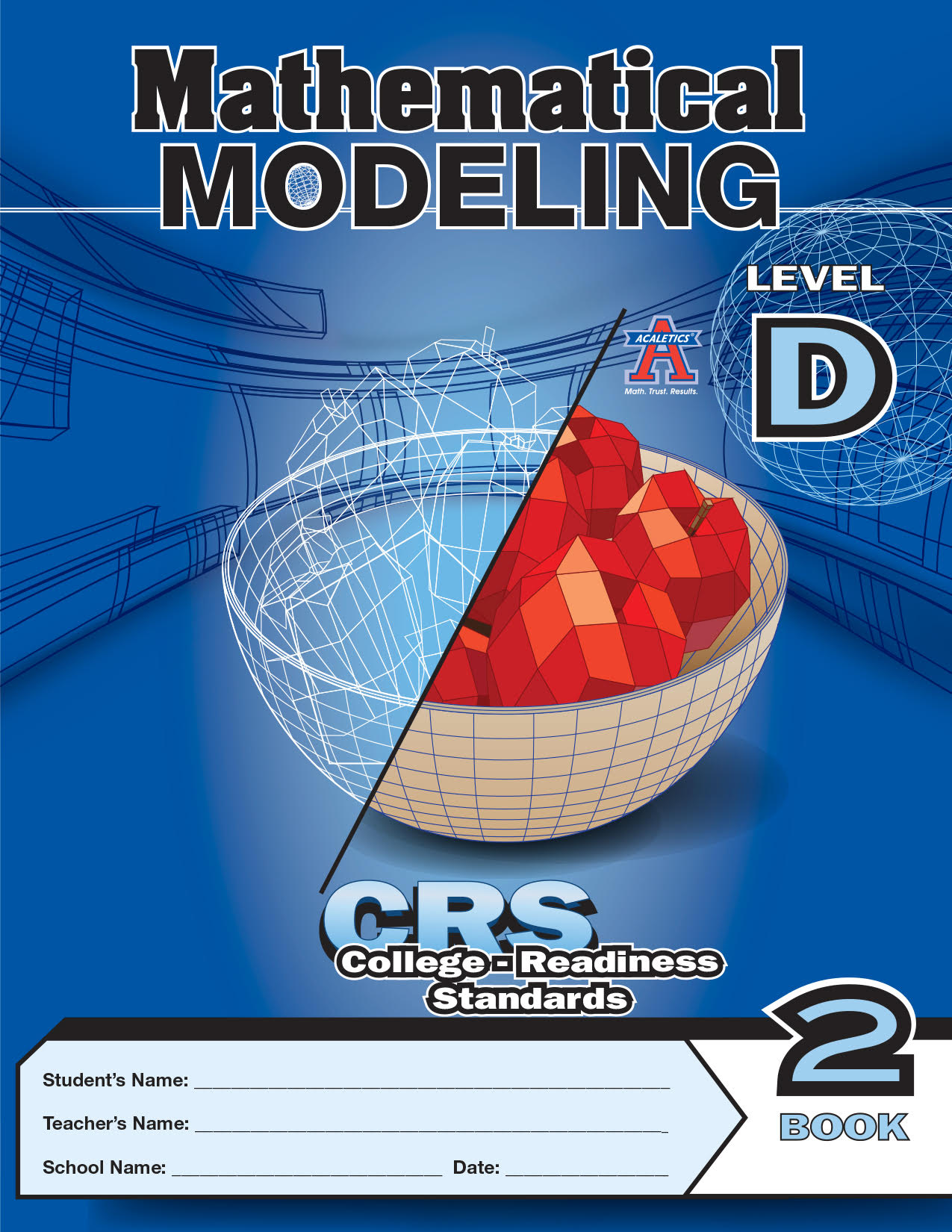close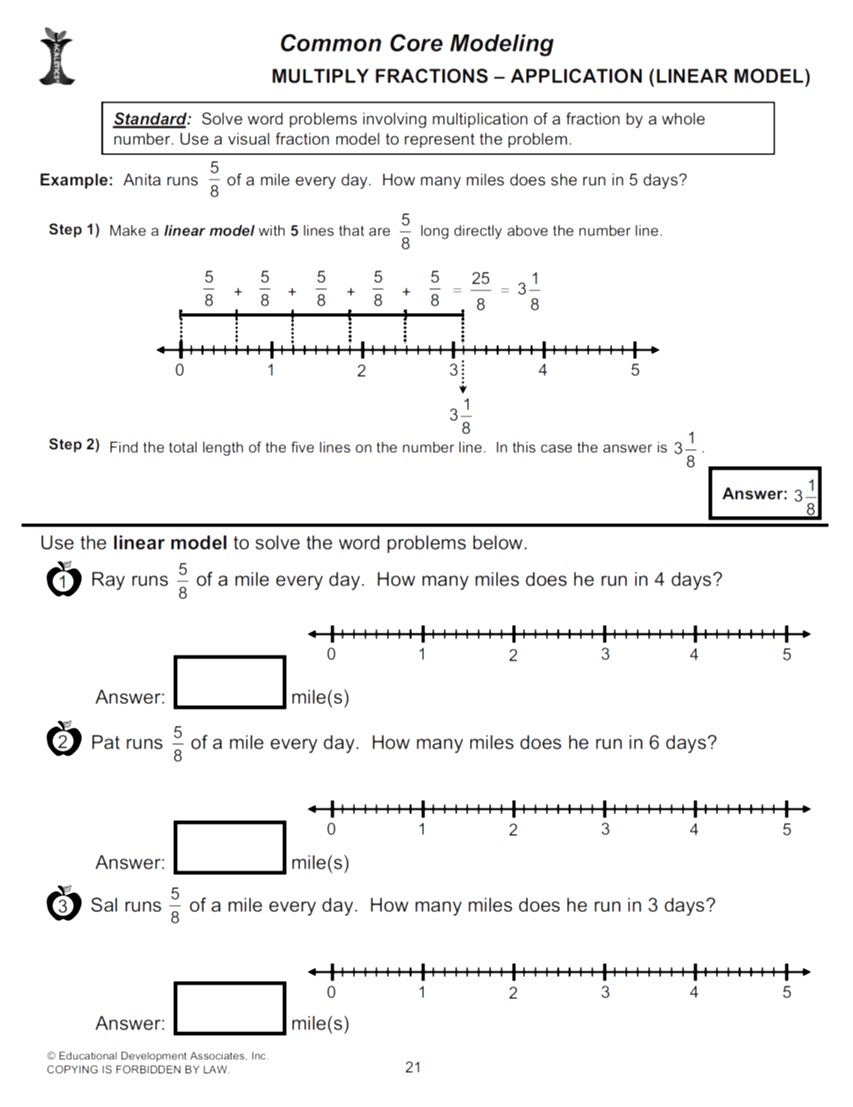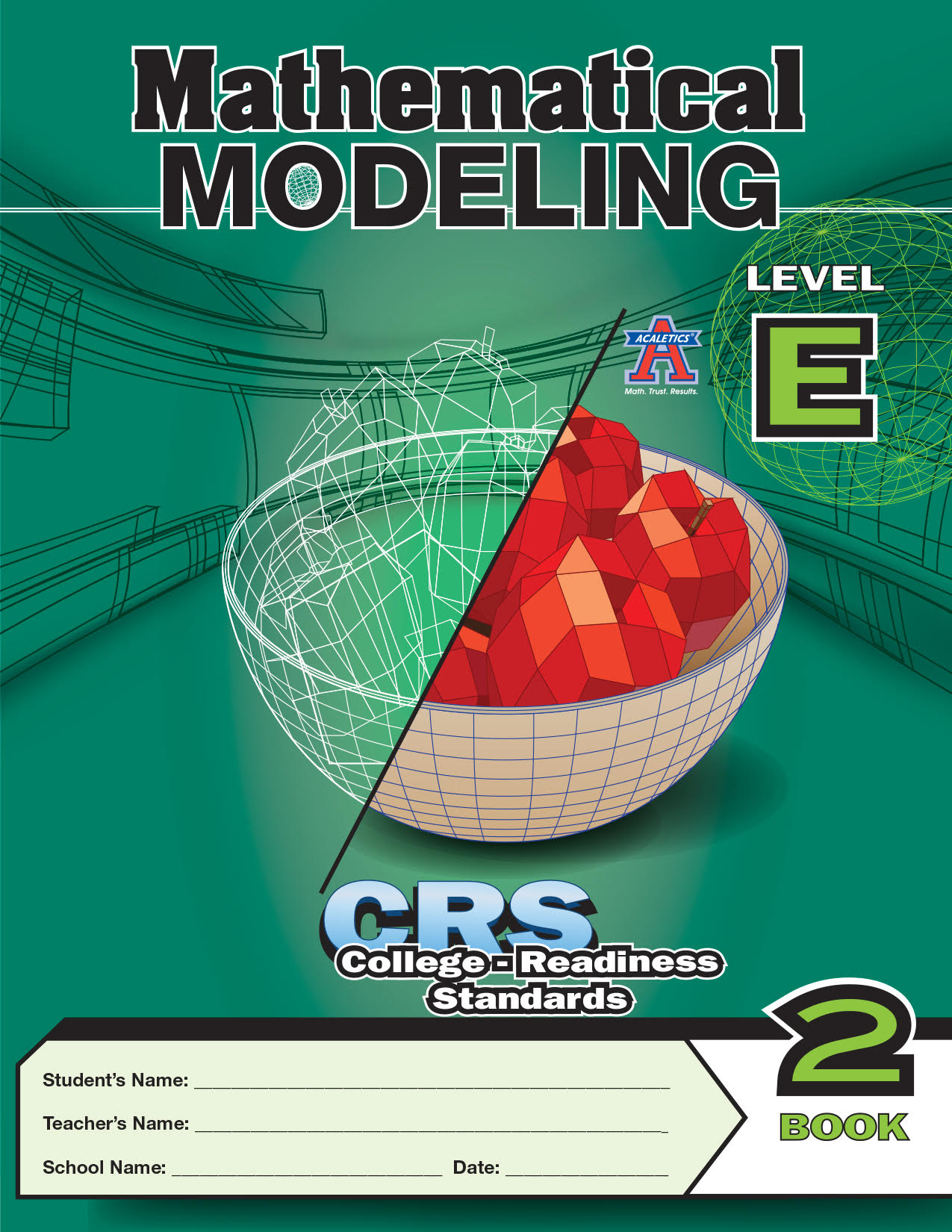close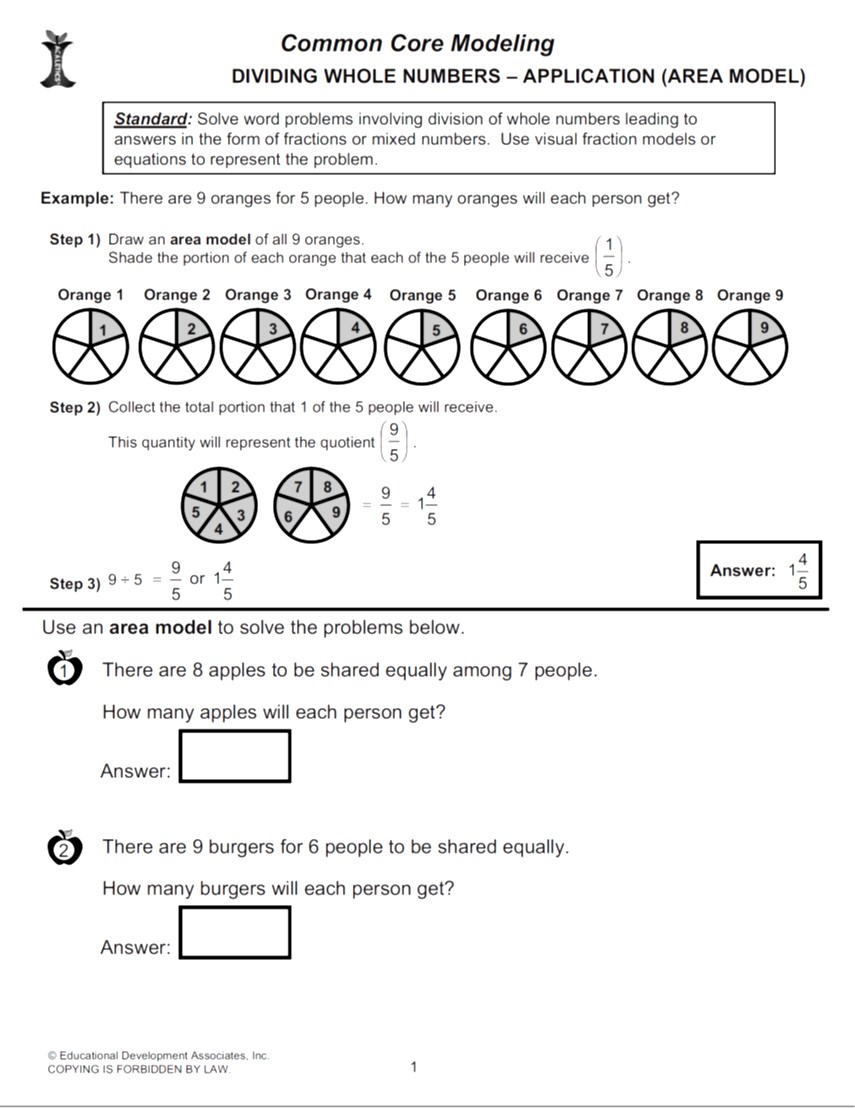This supplemental resource is the first of its kind that is solely dedicated to Mathematical Modeling!

The Mathematical Modeling Books are designed to facilitate teaching and learning of essential common core state standards concepts and skills through mathematical models. This strategy increases the student's ability to understand and solve problems by choosing and using models that represent, justify, and/or explain problems and solutions, strengthening conceptual understanding.

For students to become mathematically proficient, they must be able to experiment with representing problems in multiple ways including numbers, mathematical language (words), drawing pictures, using objects, making a chart, list, graph, or creating equations. Students need opportunities to gain conceptual understanding of these representations (models) to choose and use them appropriately.

ACALETICS® Mathematical Modeling Books for Grades 3-5 cover a number of Common Core State Standards at each grade level. These books are ideal for supplementing class work, Math ClubSM, and HomePrepSM.

For more information, contact ACALETICS® at 1-866-877-1222.